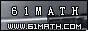Today is . WELCOME: huohai3983/44663  Home | Reg Login | All user | setHomePage | BookmarkHome | Math Test Online | Addition | Subtraction | Multiplication | Division | Time | Worksheet(JPG/PDF) | CAI | Flash Games |Recommendation articleArticle searchHome--maths--Third Grade--Subtraction Equations - Two Digit Numbers

Subtraction Equations - Two Digit Numbers

Subtraction equations - 2 digit

An equation is a mathematical statement such that the expression on the left side of the equals sign (=) has the same value as the expression on the right side. An example of an equation is 60 - 40 = 20.

One of the terms in an equation may not be know and needs to be determined. Often this unknown term is represented by a letter such as x. (e.g. x - 40 = 20).

The solution of an equation is finding the value of the unknown x. To find the value of x we can use the additive equation property which says: The two sides of an equation remain equal if the same number is added to each side.

Example:
x - 50 = 70
x - 50 + 50 = 70 + 50
x - 0 = 120
x = 120
Check the answer by substituting the value of x (120) back into the equation.
120 - 50 = 70

#### What number would complete the sentence?

 -     =

2006-12-14 22:44:16
Viewed 1892 times CloseGreatmathsites